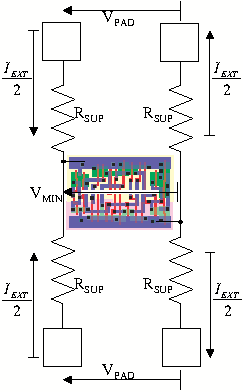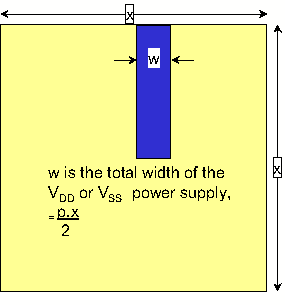The on-chip voltage drop, ΔV, is the difference between the minimum voltage at the edge of the core, Vcore, and the voltage at the centre of the core Vmin.

Referring to the drawing on the right and considering the currents and resistances of one metal layer n,

 Vcore−Vmin = ΔV = Imin×Rsup + Imin×Rsup 2 2 2

The term (Imin×Rsup)⁄2 comes from the current being supplied from two sides of the core. The overall division by 2 of the Imin voltage drops is caused by the distributed nature of the current consumption,

 so that ΔV = Imin×Rsup . 2

We prefer to work in conductances as these sum in a simple way as more metal layers are added. We say that the power supply conductance for metal layer n when the whole layer is used for power supply routing is G(n). If fraction p is used for power, then this has conductance pG(n) and

 ΔV = Imin = Imin×Rsup , and pG(n) 2 pG(n) = 2 Rsup

We refer to the second drawing on the right to derive a value for Rsup. A metal wire with length l, width w and resistivity r Ω per sq., will have a resistance

 R = l × r . w

For a core of side x, the wire length is x⁄2. The wire width is the fraction p of the metal layer used for power divided by 2 for two power supplies Vdd and Vss. The length and width of each power supply is

 l = x , w = px . 2 2

The power supply resistance for metal layer n is then

 Rsup = 2x × rn = rn (independent of the size of the core) 2px p
and the conductance
 pG(n) = 2 = 2p , and Rsup rn

 G(n) = 2 rn

This discussion assumes that all the power straps carry current from the edge to the centre of the core. In fact though, there is a manhattan grid in x and y which means that many of the power straps contribute less useful current. The effect of this is modelled with a derating. The value is found by using Spice to model a supply strap grid and measuring the voltage drop with an electrical simulation.

The derating number for the conductance from the simulation is 7⁄8, which gives the expression for the supply conductance of metal layer n

 G(n) = 2 × 7 = 7 and Gsup = ∑G(n) rn 8 4×rnPicture showing the current flows and resistances for the calculation of on-chip IR drop. Vpad is the same as Vcore and Iext is the same as Imin.Picture to illustate the derivation of the resistance of a supply line carrying current from the core edge to the centre.